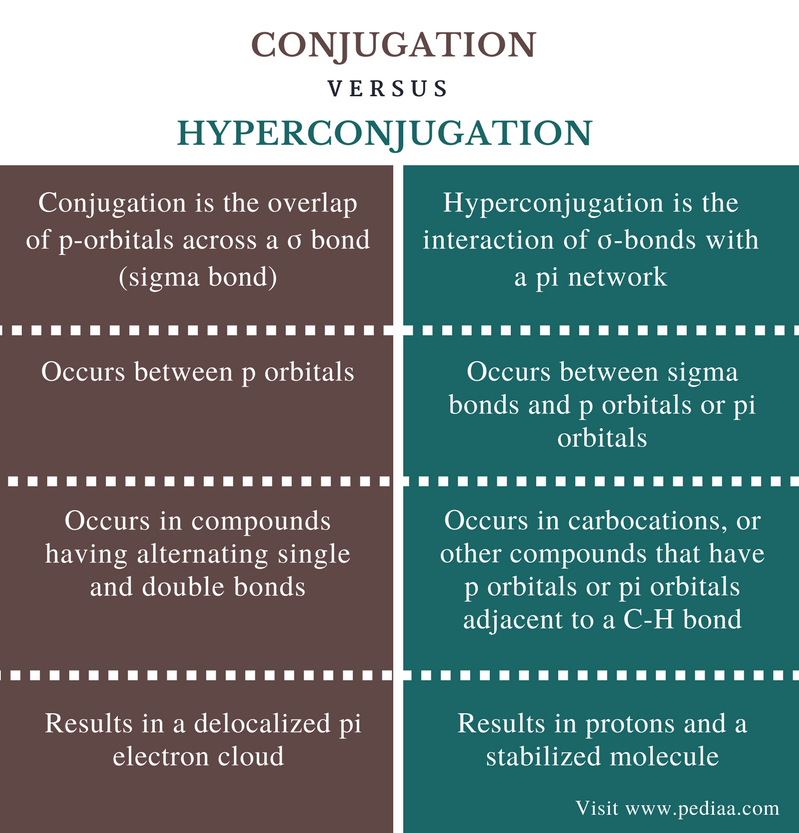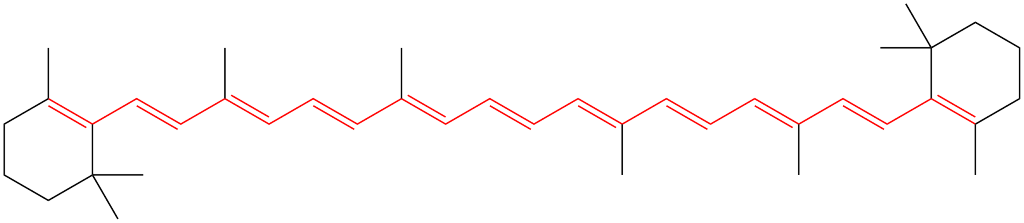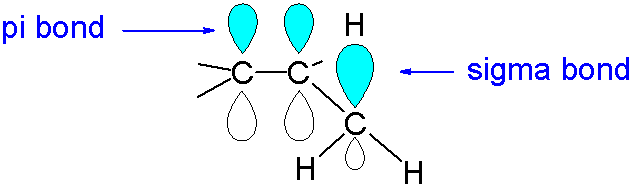# Difference Between Conjugation and Hyperconjugation

## Main Difference – Conjugation vs Hyperconjugation

The terms conjugation and hyperconjugation are related to unsaturated organic compounds. The term conjugation has different meanings in chemistry; conjugation may refer to the joining of two compounds to form a single compound or it can be the overlap of p-orbitals across a σ bond (sigma bond). Since we are comparing conjugation with hyperconjugation, i.e., interaction of σ-bonds with a π network, in this article, we’ll consider the second definition of conjugation. Thus, the main difference between conjugation and hyperconjugation is that conjugation is the overlap of p-orbitals across a σ bond whereas hyperconjugation is the interaction of σ-bonds with a pi network.

### Key Areas Covered

1. What is Conjugation
– Definition, Sigma Bonds, Mechanism
2. What is Hyperconjugation
– Definition, Explanation
3. What is the Difference Between Conjugation and Hyperconjugation
– Comparison of Key Differences

Key Term: Carbon, Carbocation, Conjugation, Hydrogen, Hyperconjugation, Orbital, Pi Bond, P Orbital, Sigma Bond## What is Conjugation

Conjugation is the overlap of p-orbitals across a σ bond (sigma bond). A sigma bond is a type of a covalent bond. Unsaturated compounds having double bonds are composed of one sigma bond and a pi bond. The carbon atoms of these compounds are sp2 hybridized. Since the hybridization is sp2, there is an un-hybridized p orbital per each carbon atom. When a compound has alternating single bonds (sigma bonds) and double bonds (a sigma bond and a pi bond), the un-hybridized p orbitals can get overlapped with each other, forming an electron cloud. The electrons in those p orbitals then get delocalized inside this electron cloud. This kind of delocalized system is known as a conjugated system. Hence, this overlapping of p orbitals is known as conjugation.Figure 1: Beta-Carotene-Conjugated System

A sigma bond is a strong covalent bond that is formed due to a head-on mixing between two atomic orbitals. The simplest covalent bonds are formed between two s orbitals of two atoms. But in atoms with complex atomic structures, atomic orbitals undergo hybridization (mixing of atomic orbitals to form hybrid orbitals having new shapes). Sp2 hybridization is the hybridization between one s orbital and two p orbitals. Since an atom has three p orbitals, an un-hybridized p orbital remains after sp2 hybridization. If all adjacent carbon atoms of a compound have un-hybridized p orbitals, these orbitals can overlap with each other. This creates a conjugated system.

Conjugation can be observed in aromatic compounds, which are also cyclic compounds. Benzene is an aromatic compound having a conjugated pi electron system. The benzene ring is made of six carbon atoms that are sp2 hybridized. Therefore, all six carbon atoms have un-hybridized p orbitals. These orbitals overlap with each other, forming a conjugated system.

## What is Hyperconjugation

Hyperconjugation is the interaction of σ-bonds with a pi network. Here, electrons in a sigma bond interact with an adjacent partially (or completely) filled p orbital, or with a pi orbital. Hyperconjugation occurs to increase the stability of a molecule.Figure 2: Hyperconjugation Can Occur between a Pi Orbital and a Sigma Bond

A hyperconjugation is caused by the overlap of bonding electrons in C-H sigma bond with a p orbital or a pi orbital of the adjacent carbon atom. The hydrogen atom resides in a close proximity as a proton. The negative charge that develops on the carbon atom is delocalized due to the overlap of p orbital or pi orbital.

There are several effects of hyperconjugation on chemical properties of compounds. For example, in carbocation, hyperconjugation causes the positive charge on the carbon atom.

## Difference Between Conjugation and Hyperconjugation

### Definition

Conjugation: Conjugation is the overlap of p-orbitals across a σ bond (sigma bond).

Hyperconjugation: Hyperconjugation is the interaction of σ-bonds with a pi network.

### Components Involved

Conjugation: Conjugation occurs between p orbitals.

Hyperconjugation: Hyperconjugation occurs between sigma bonds and p orbitals or pi orbitals.

### Occurrence

Conjugation: Conjugation occurs in compounds having alternating single and double bonds.

Hyperconjugation: Hyperconjugation occurs in carbocations or other compounds that have p orbitals or pi orbitals adjacent to a C-H bond.

### Outcome

Conjugation: Conjugation results in a delocalized pi electron cloud.

Hyperconjugation: Hyperconjugation results in protons and a stabilized molecule.

### Conclusion

The two terms conjugation and hyperconjugation describe unsaturated organic compounds. The main difference between conjugation and hyperconjugation is that conjugation is the overlap of p-orbitals across a σ bond whereas hyperconjugation is the interaction of σ-bonds with a pi network.

##### Reference:

1. Helmenstine, Anne Marie, Ph.D. “Conjugate Definition in Chemistry.” ThoughtCo, Mar. 19, 2017, Available here.
2. “Conjugated system.” Wikipedia, Wikimedia Foundation, 26 Jan. 2018, Available here.
3. Devyani Joshi, Trainee at SRS Pharmaceuticals Pvt. Ltd., India Follow. “Hyperconjugation – organic chemistry.” LinkedIn SlideShare, 10 Nov. 2016, Available here.

##### Image Courtesy:

1. “Beta-carotene-conjugation” By Sirjasonr – Own work (Public Domain) via Commons Wikimedia
2. “HyperconjugationInSubstitutedAlkenes” By V8rik at the English language Wikipedia (CC BY-SA 3.0) via Commons Wikimedia